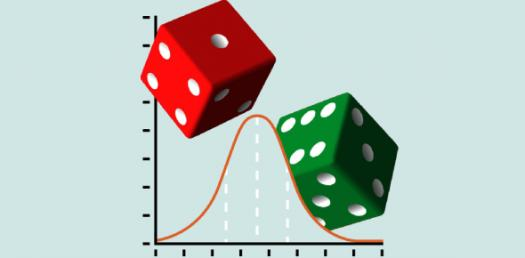# Take The Quiz To Test Your Probability Knowledge!

15 Questions | Attempts: 9802
ShareSettingsWhat do you know about probability? When faced with a problem such as whether the coin you flipped will land on heads or tails, you need first to identify the number of possible outcomes. For any event, every possible outcome has to be identified first. Identify the number of favorable outcomes. When all the numbers are attained, calculate the probability. Take this quiz to test your probability knowledge.

• 1.
In a class, there are 12 boys and 16 girls. One of them is called out by an enroll number, what is the probability that the one called is a girl?
• A.

1/4

• B.

2/5

• C.

5/12

• D.

4/7

• 2.
There are 12 boys and 8 girls in a tution centre. If three of them scored first mark, then what is the probability that one of the three is a girl and the other two are boys?
• A.

14/75

• B.

22/55

• C.

44/95

• D.

None of these

• 3.
Two dice are thrown simultaneously. What is the probability of getting two numbers whose product is even?
• A.

1/2

• B.

3/4

• C.

3/8

• D.

5/16

• 4.
From a pack of 52 cards, two cards are drawn together at random. What is the probability of both the cards being kings?
• A.

1/15

• B.

25/57

• C.

1/221

• D.

35/256

• 5.
Two dice are tossed. The probability that the total score is a prime number is:
• A.

1/6

• B.

5/12

• C.

1/2

• D.

7/9

• 6.
Three unbiased coins are tossed. What is the probability of getting at most two heads?
• A.

3/4

• B.

1/4

• C.

3/8

• D.

7/8

• 7.
A card is drawn from a pack of 52 cards. The probability of getting a queen of club or a king of heart is:
• A.

1/26

• B.

1/13

• C.

2/26

• D.

2/13

• 8.
A bag contains 7 blue balls and 5 yellow balls. If two balls are selected at random, what is the probability that none is yellow?
• A.

5/33

• B.

7/22

• C.

7/33

• D.

5/22

• 9.
A bag contains four blue, three green and five red balls.If three balls are drawn at random from the bag, then what is the probability that all are red?
• A.

1/11

• B.

1/22

• C.

3/11

• D.

3/22

• 10.
A bag contains four blue, three green and five red balls.If four balls are drawn at random, what is the probability that two are blue and two are green?
• A.

2/55

• B.

1/55

• C.

1/22

• D.

None of the above

• 11.
A bag contains four blue, three green and five red ballsIf three balls are drawn at random, what is the probability that all the three balls are of different colours?
• A.

1/11

• B.

2/11

• C.

3/11

• D.

4/11

• 12.
A bag contains four blue, three green and five red balls.If three balls are drawn at random, what is the probability that three is at least one red ball in the three balls?
• A.

37/44

• B.

31/44

• C.

19/22

• D.

13/22

• 13.
If 3 cards are drawn simultaneously form a pack of well shuffled cards, find the probability of then being all Queen.
• A.

26/221

• B.

143/110504

• C.

4/13

• D.

1/2

• E.

None of these

• 14.
In a shooting test, the probability of hitting the target is 1/2 for A and 2/3 for B and 3/4 for C. Find the probability that the target is hit
• A.

1/24

• B.

23/24

• C.

1/4

• D.

None of these

• 15.
In a shooting test, the probability of hitting the target is 1/2 for A and 2/3 for B and 3/4 for C. Find the probability that all of them hit the target.
• A.

1/24

• B.

23/24

• C.

1/4

• D.

None of these

## Related TopicsBack to top
×

Wait!
Here's an interesting quiz for you.